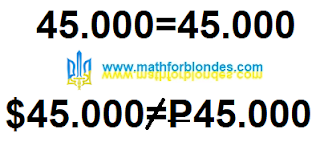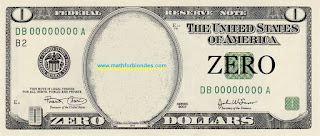## 2/21/2023

### Where does math end?

I was offered to sell my Russian site for 45.000 rubles. This prompted me to a very interesting question: "Where does mathematics end?". And here's my answer: "Math ends where money begins". Try any mathematical theory to apply to money and you will learn a lot of interesting things about this theory.

I love math trolling. The sentence contained the phrase: "The price may be revised upwards". My response was: "\$45.000 Price may be revised down". From the point of view of mathematics, the equality 45.000=45.000 is beyond doubt. As soon as I add different units of money to this equality, it disappears.Where does math end?

We use numbers to measure money. Numbers are written on coins and banknotes. Have you heard of banknote or a coin with the number "zero"? Zero is not a number. The more I study this question, the more I am convinced of the correctness of my statement.Zero dollars

I'm not even talking about the minus sign on money. Through the prism of money, I considered set theory. The result is very interesting.